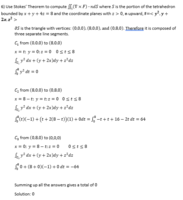# Stokes' Theorem

#### burt

##### Junior MemberThis is the problem I just worked through. My problem is that, although I can't see any error, it seems to have worked out to neatly. Is this right?

Also, although I just did the work, I don't really understand what it means. If the answer is zero, does that mean the flux of a fluid on this vector field is zero? Meaning that it is not moving at all? Or that the same exact amount is coming out as is going in?

#### HallsofIvy

##### Elite Member
Going from (8, 0, 0) to (0, 8, 0) you have x= 8- t, y= t so dx= -dt and the first term is $$\displaystyle \int y^2 dx= t^2(-dt)$$ but you have only $$\displaystyle \int t (-dt)$$.

•burt